# Case Injected Genetic Algorithms Sushil J Louis Genetic

• Slides: 39Case Injected Genetic Algorithms Sushil J. Louis Genetic Algorithm Systems Lab (gaslab) University of Nevada, Reno http: //www. cs. unr. edu/~sushil http: //gaslab. cs. unr. edu/ [email protected] unr. eduOutline w Motivation w What is the technique? n Genetic Algorithm and Case-Based Reasoning w Is it useful? n Evaluate performance on Combinational Logic Design w Results w Conclusions http: //gaslab. cs. unr. eduOutline w Motivation w What is the technique? n Genetic Algorithm and Case-Based Reasoning w Is it useful? n n Combinational Logic Design Strike Force Asset Allocation TSP Scheduling w Conclusions http: //gaslab. cs. unr. edu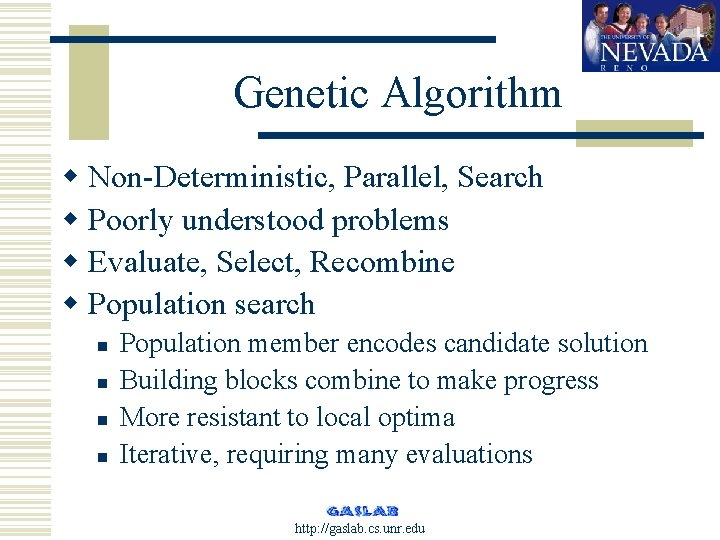Genetic Algorithm w Non-Deterministic, Parallel, Search w Poorly understood problems w Evaluate, Select, Recombine w Population search n n Population member encodes candidate solution Building blocks combine to make progress More resistant to local optima Iterative, requiring many evaluations http: //gaslab. cs. unr. eduMotivation w Deployed systems are expected to confront and solve many problems over their lifetime w How can we increase genetic algorithm performance with experience? w Provide GA with a memory http: //gaslab. cs. unr. eduCase-Based Reasoning w When confronted by a new problem, adapt similar (already solved) problem’s solution to solve new problem w CBR Associative Memory + Adaptation w CBR: Indexing (on problem similarity) and adaptation are domain dependent http: //gaslab. cs. unr. edu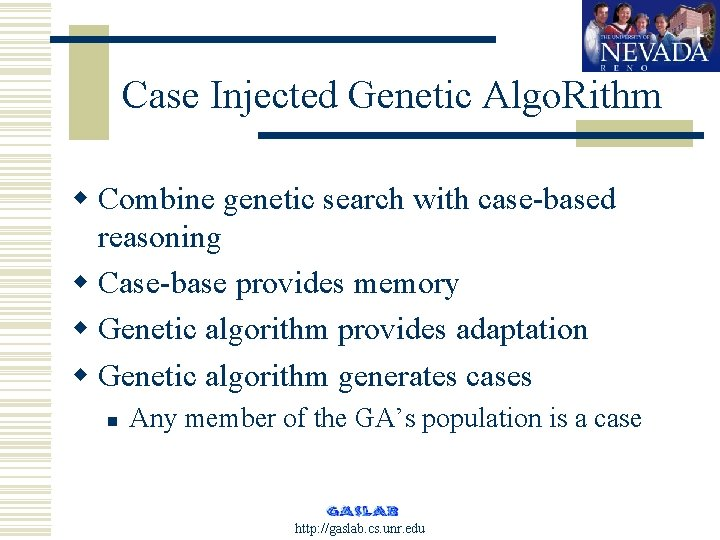Case Injected Genetic Algo. Rithm w Combine genetic search with case-based reasoning w Case-base provides memory w Genetic algorithm provides adaptation w Genetic algorithm generates cases n Any member of the GA’s population is a case http: //gaslab. cs. unr. eduSystem http: //gaslab. cs. unr. eduRelated work w Seeding: Koza, Greffensttette, Ramsey, Louis w Lifelong learning: Thrun w Key Differences n n Store and reuse intermediate solutions Solve sequences of similar problems http: //gaslab. cs. unr. eduCombinational Logic Design w An example of configuration design w Given a function and a target technology to work with design an artifact that performs this function subject to constraints n n n Target technology: Logic gates Function: Parity checking Constraints: 2 -D gate array http: //gaslab. cs. unr. eduEncoding http: //gaslab. cs. unr. eduEncoding http: //gaslab. cs. unr. edu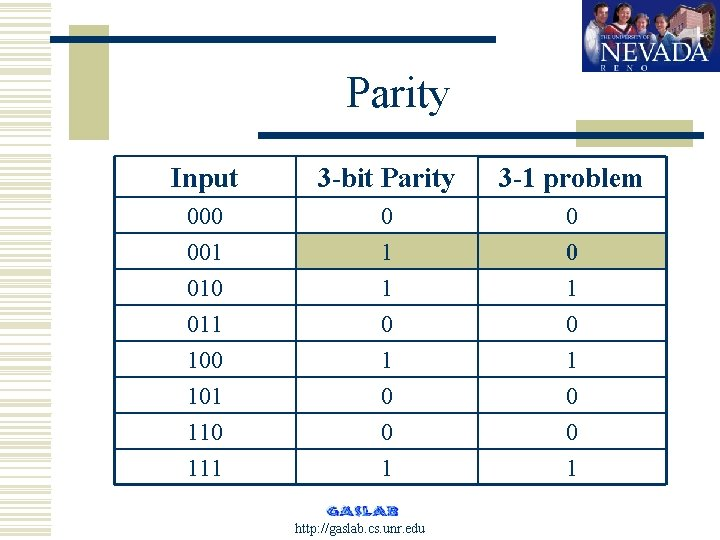Parity Input 3 -bit Parity 3 -1 problem 000 001 010 011 100 101 110 111 0 1 0 0 1 http: //gaslab. cs. unr. eduWhich cases to inject? w Problem distance metric (Louis ‘ 97) n Domain dependent w Solution distance metric n Genetic algorithm encodings Binary – hamming distance l Real – euclidean distance l Permutation – longest common substring l… l http: //gaslab. cs. unr. eduProblem similarity http: //gaslab. cs. unr. eduSolving parity problem after solving parity-8 w Inject solution to parity 6 -8 into population of ga solving parity 6 n Storing and Injecting solutions may not improve solution quality w Inject a solution from a prior generation of GA solving parity-8 n Storing and Injecting partial solutions does lead to improved quality http: //gaslab. cs. unr. eduInjecting earlier (partial) solutions on OSSP http: //gaslab. cs. unr. edu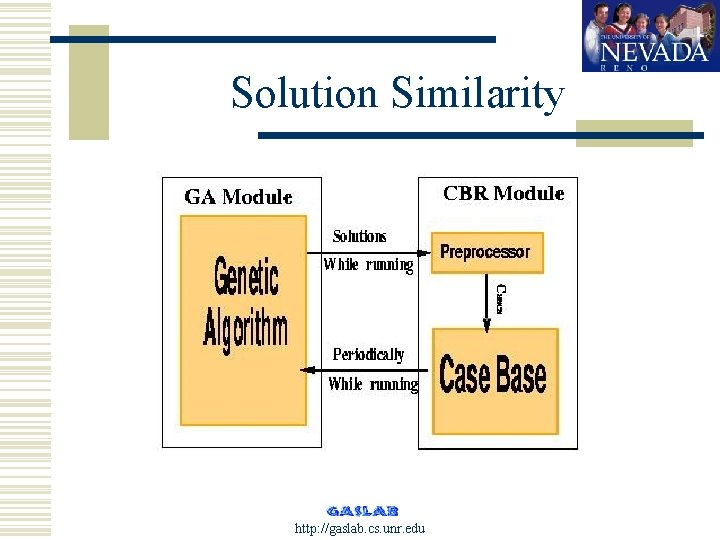Solution Similarity http: //gaslab. cs. unr. edu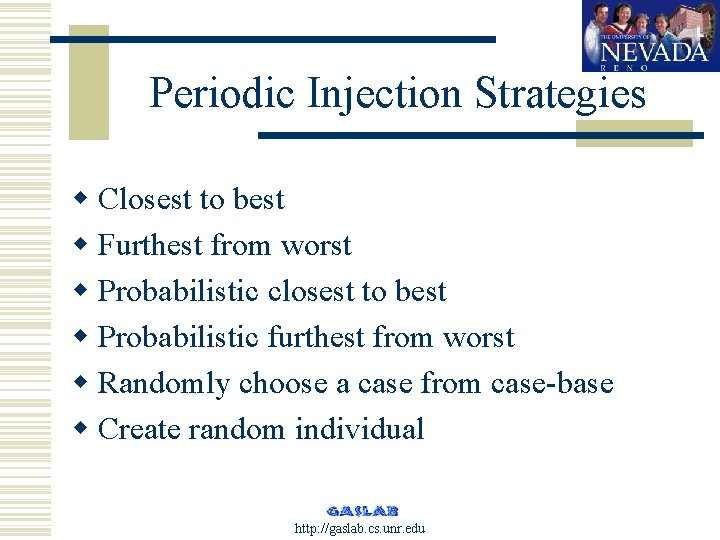Periodic Injection Strategies w Closest to best w Furthest from worst w Probabilistic closest to best w Probabilistic furthest from worst w Randomly choose a case from case-base w Create random individual http: //gaslab. cs. unr. edu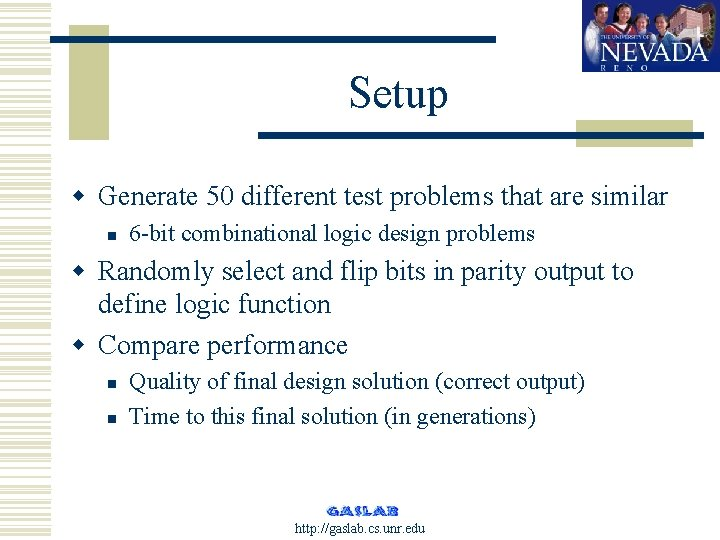Setup w Generate 50 different test problems that are similar n 6 -bit combinational logic design problems w Randomly select and flip bits in parity output to define logic function w Compare performance n n Quality of final design solution (correct output) Time to this final solution (in generations) http: //gaslab. cs. unr. edu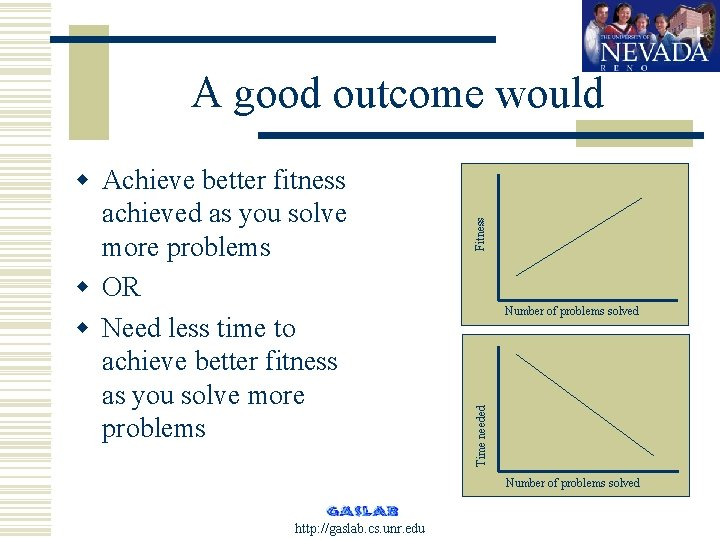Number of problems solved Time needed w Achieve better fitness achieved as you solve more problems w OR w Need less time to achieve better fitness as you solve more problems Fitness A good outcome would Number of problems solved http: //gaslab. cs. unr. edu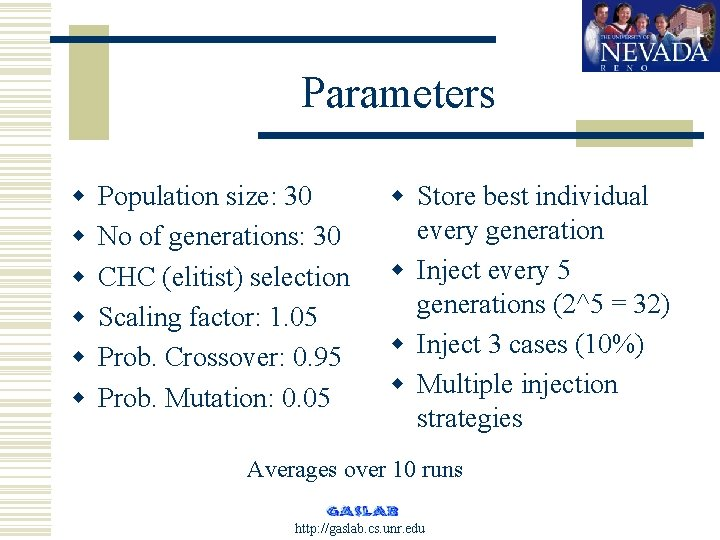Parameters w w w Population size: 30 No of generations: 30 CHC (elitist) selection Scaling factor: 1. 05 Prob. Crossover: 0. 95 Prob. Mutation: 0. 05 w Store best individual every generation w Inject every 5 generations (2^5 = 32) w Inject 3 cases (10%) w Multiple injection strategies Averages over 10 runs http: //gaslab. cs. unr. eduProblem distribution http: //gaslab. cs. unr. edu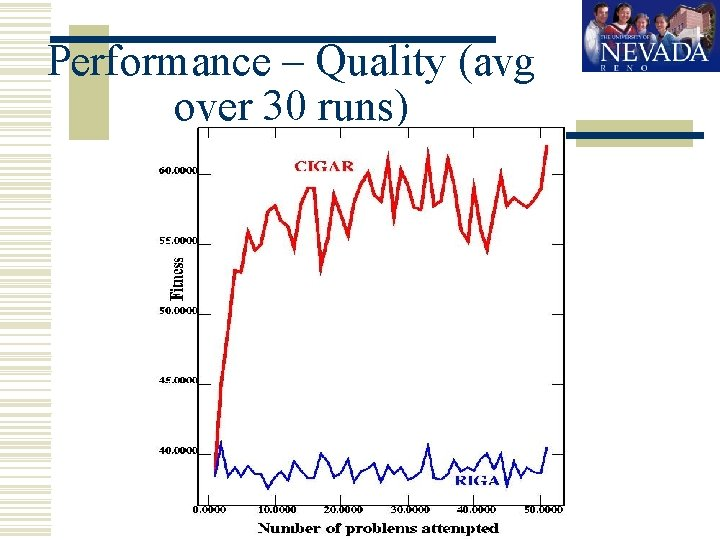Performance – Quality (avg over 30 runs) http: //gaslab. cs. unr. eduPerformance - Time http: //gaslab. cs. unr. eduInjection Strategies http: //gaslab. cs. unr. edu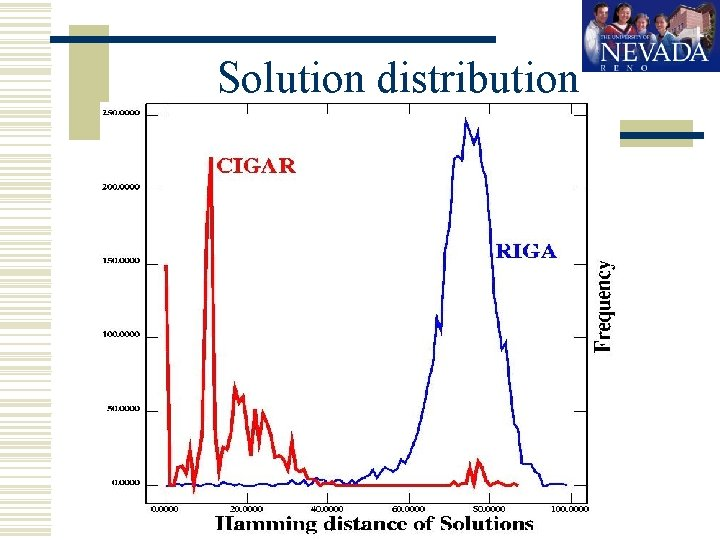Solution distribution http: //gaslab. cs. unr. eduStrike force asset allocation w Allocate platforms to targets w Dynamic n n n Changing Priority Battlefield conditions Popup Weather … http: //gaslab. cs. unr. edu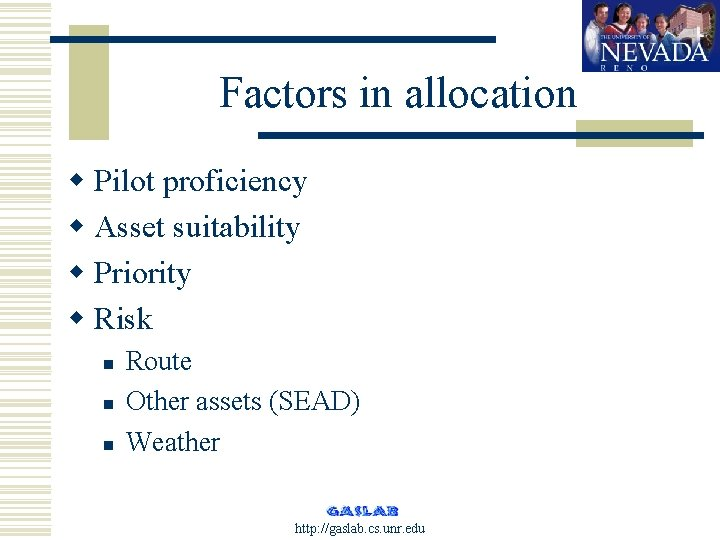Factors in allocation w Pilot proficiency w Asset suitability w Priority w Risk n n n Route Other assets (SEAD) Weather http: //gaslab. cs. unr. edu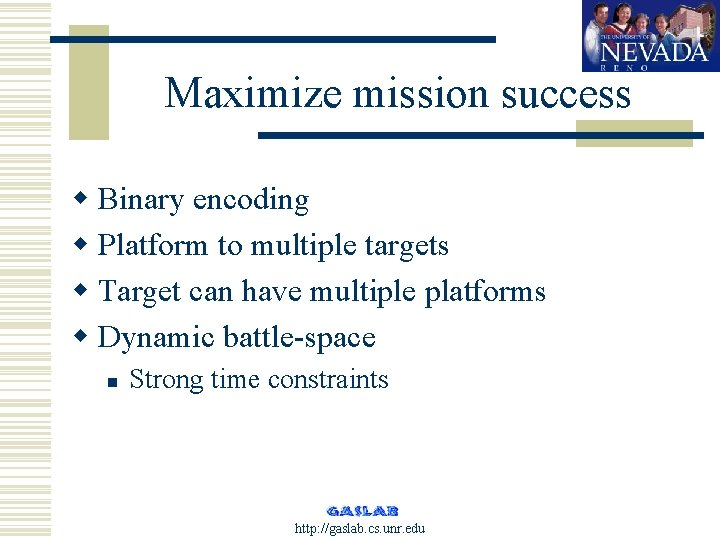Maximize mission success w Binary encoding w Platform to multiple targets w Target can have multiple platforms w Dynamic battle-space n Strong time constraints http: //gaslab. cs. unr. edu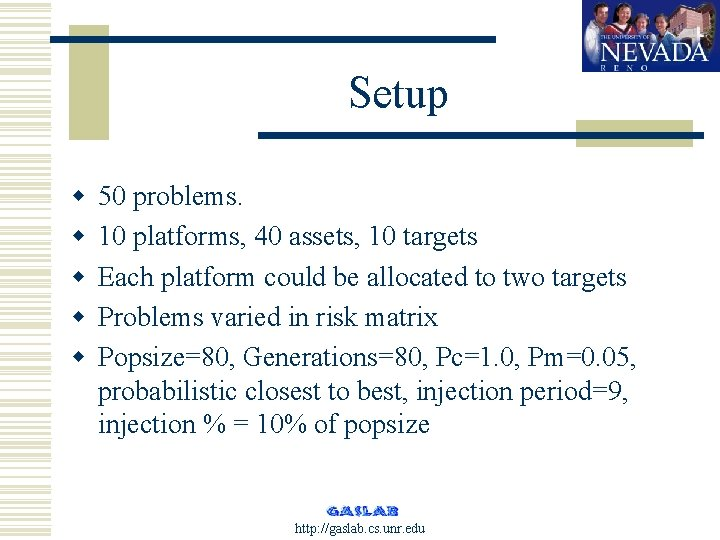Setup w w w 50 problems. 10 platforms, 40 assets, 10 targets Each platform could be allocated to two targets Problems varied in risk matrix Popsize=80, Generations=80, Pc=1. 0, Pm=0. 05, probabilistic closest to best, injection period=9, injection % = 10% of popsize http: //gaslab. cs. unr. edu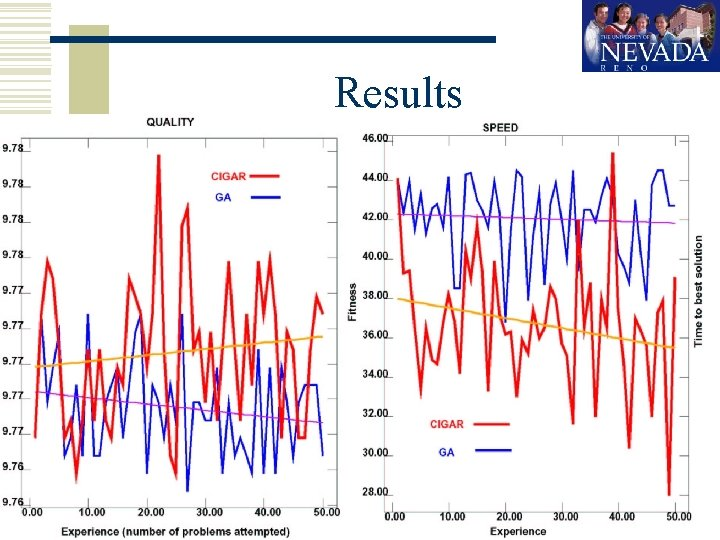Results http: //gaslab. cs. unr. edu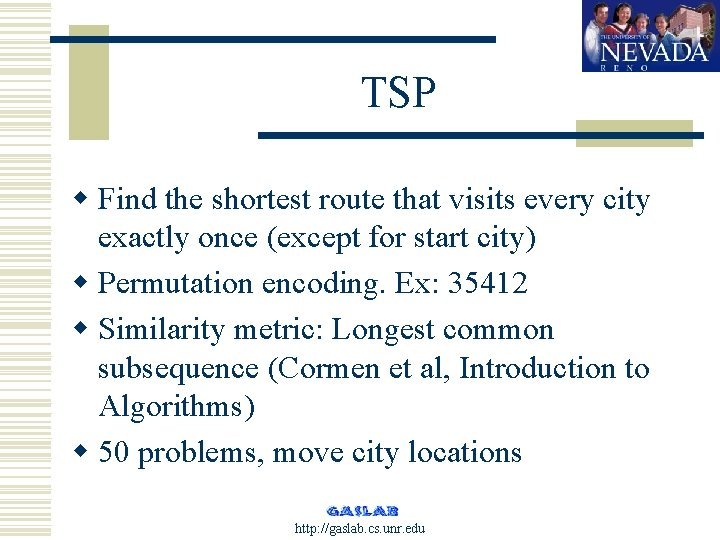TSP w Find the shortest route that visits every city exactly once (except for start city) w Permutation encoding. Ex: 35412 w Similarity metric: Longest common subsequence (Cormen et al, Introduction to Algorithms) w 50 problems, move city locations http: //gaslab. cs. unr. edu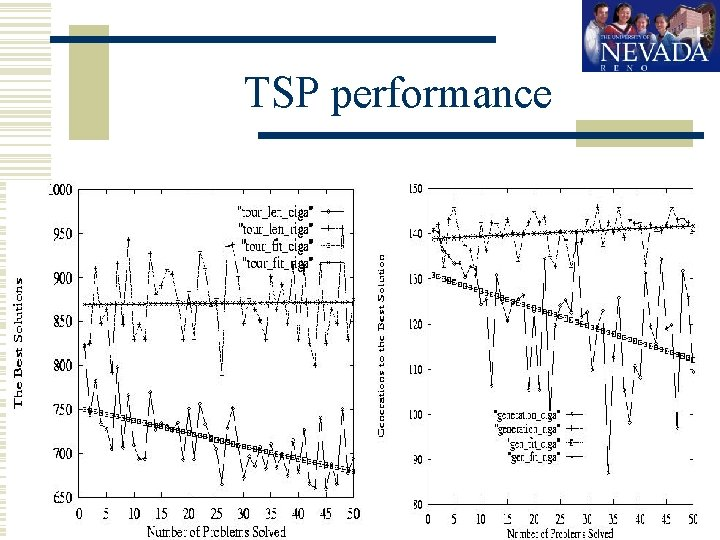TSP performance http: //gaslab. cs. unr. edu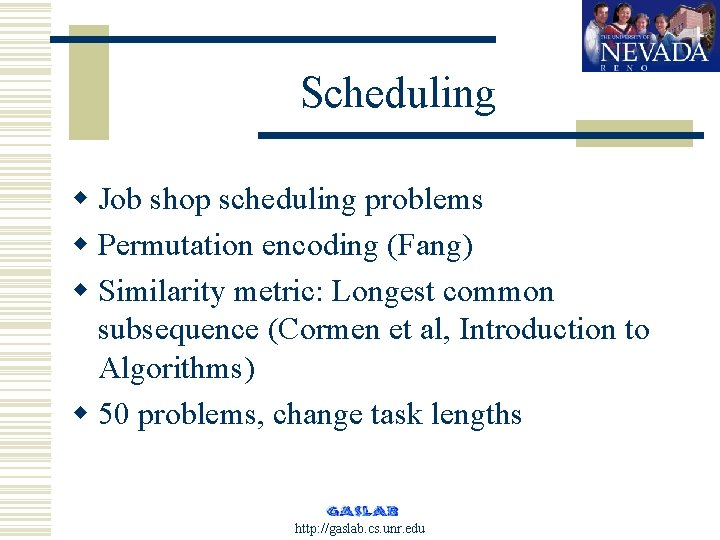Scheduling w Job shop scheduling problems w Permutation encoding (Fang) w Similarity metric: Longest common subsequence (Cormen et al, Introduction to Algorithms) w 50 problems, change task lengths http: //gaslab. cs. unr. edu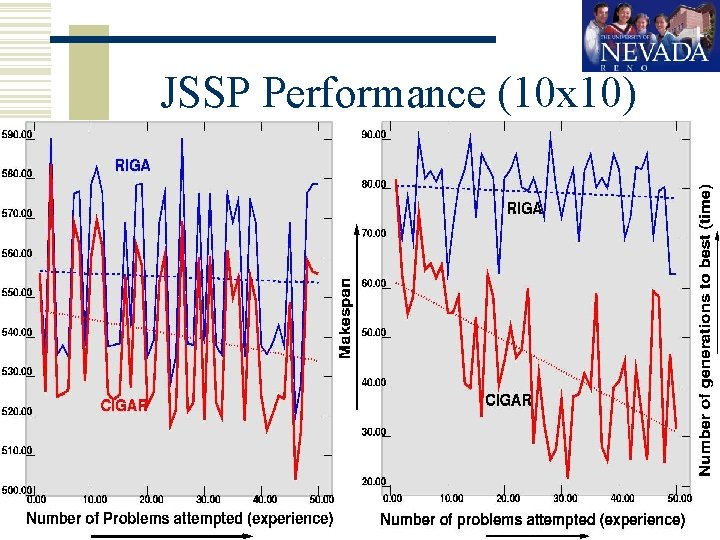JSSP Performance (10 x 10) http: //gaslab. cs. unr. eduJSSP Performance (15 x 15) http: //gaslab. cs. unr. edu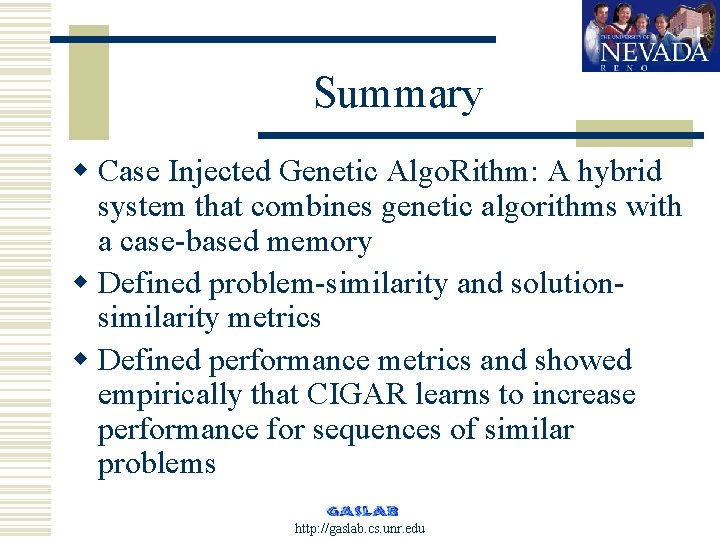Summary w Case Injected Genetic Algo. Rithm: A hybrid system that combines genetic algorithms with a case-based memory w Defined problem-similarity and solutionsimilarity metrics w Defined performance metrics and showed empirically that CIGAR learns to increase performance for sequences of similar problems http: //gaslab. cs. unr. edu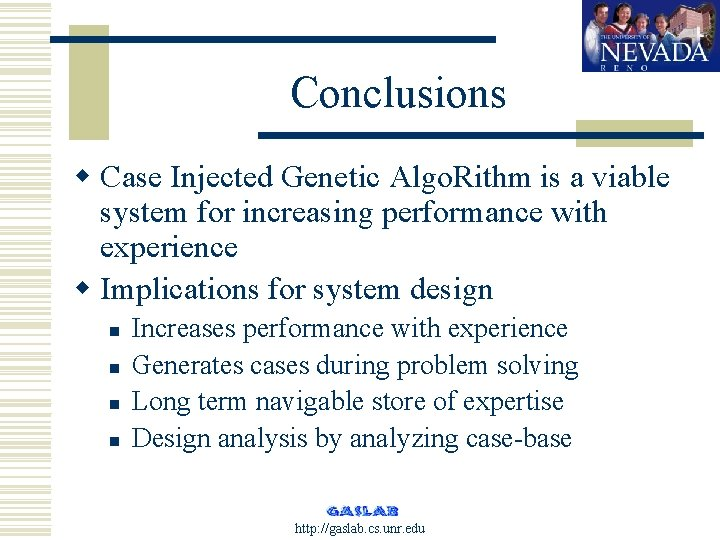Conclusions w Case Injected Genetic Algo. Rithm is a viable system for increasing performance with experience w Implications for system design n n Increases performance with experience Generates cases during problem solving Long term navigable store of expertise Design analysis by analyzing case-base http: //gaslab. cs. unr. edu